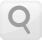# CASIO

User´s Guide## Calculation Ranges, Number of Digits, and Precision

The calculation range, number of digits used for internal calculation, and calculation precision depends on the type of calculation you are performing.

### Calculation Range and Precision

Calculation Range ±1 × 10-99 to ±9.999999999 × 1099 or 0 15 digits In general, ±1 at the 10th digit for a single calculation. Precision for exponential display is ±1 at the least significant digit. Errors are cumulative in the case of consecutive calculations.

### Function Calculation Input Ranges and Precision

Functions Input Range
sinx
cosx
Deg 0 ≦ |x| < 9 × 109
Rad 0 ≦ |x| < 157079632.7
Gra 0 ≦ |x| < 1 × 1010
tanx Deg Same as sinx, except when |x| = (2n-1) × 90.
Rad Same as sinx, except when |x| = (2n-1) × π/2.
Gra Same as sinx, except when |x| = (2n-1) × 100.
sin-1x, cos-1x 0 ≦ |x| ≦ 1
tan-1x 0 ≦ |x| ≦ 9.999999999 × 1099
sinhx, coshx 0 ≦ |x| ≦ 230.2585092
sinh-1x 0 ≦ |x| ≦ 4.999999999 × 1099
cosh-1x 1 ≦ x ≦ 4.999999999 × 1099
tanhx 0 ≦ |x| ≦ 9.999999999 × 1099
tanh-1x 0 ≦ |x| ≦ 9.999999999 × 10-1
logx, lnx 0 < x ≦ 9.999999999 × 1099
10x -9.999999999 × 1099x ≦ 99.99999999
ex -9.999999999 × 1099x ≦ 230.2585092
x 0 ≦ x < 1 × 10100
x2 |x| < 1 × 1050
x-1 |x| < 1 × 10100 ; x ≠ 0
3x |x| < 1 × 10100
x! 0 ≦ x ≦ 69 (x is an integer)
nPr 0 ≦ n < 1 × 1010, 0 ≦ rn (n, r are integers)
1 ≦ {n!/(n-r)!} < 1 × 10100
nCr 0 ≦ n < 1 × 1010, 0 ≦ rn (n, r are integers)
1 ≦ n!/r! < 1 × 10100 or 1 ≦ n!/(n-r)! < 1 × 10100
Pol(x, y) |x|, |y| ≦ 9.999999999 × 1099
x2 + y2 ≦ 9.999999999 × 1099
Rec(r, θ) 0 ≦ r ≦ 9.999999999 × 1099
θ: Same as sinx
°’ ”

°’ ”
a°bc”: |a|, b, c < 1 × 10100 ; 0 ≦ b, c
The display seconds value is subject to an error of ±1 at the second decimal place.
|x| < 1 × 10100
Decimal ↔ Sexagesimal Conversions
0°0°0° ≦ |x| ≦ 9999999°59°
xy x > 0: -1 × 10100 < ylogx < 100
x = 0: y > 0
x < 0: y = n, 12n+1 (n is an integer)
However: -1 × 10100 < ylog |x| < 100
xy y > 0: x ≠ 0, -1 × 10100 < 1/x logy < 100
y = 0: x > 0
y < 0: x = 2n+1, 1n (n ≠ 0; n is an integer)
However: -1 × 10100 < 1/x log |y| < 100
ab/c Total of integer, numerator, and denominator must be 10 digits or less (including division marks).
RanInt#(a, b) a < b ; |a|, |b| < 1 × 1010 ; b - a < 1 × 1010 (a , b are integers)

Precision is basically the same as that described under "Calculation Range and Precision", above.

Calculations that use any of the functions or settings shown below require consecutive internal calculations to be performed, which can cause accumulation of error that occurs with each calculation.
xy, xy , 3 , x!, nPr, nCr; °, r, g (Angle unit: Rad); σx, sx, regression coefficient.

Error is cumulative and tends to be large in the vicinity of a function's singular point and inflection point.

During statistical calculation, error is cumulative when data values have a large number of digits and the differences between data values is small. Error will be large when data values are greater than six digits.